Practice Problems - Page 23Custom SearchPRACTICE PROBLEMS: Change the equations in problems 1 through 4 to equations having polar coordinates.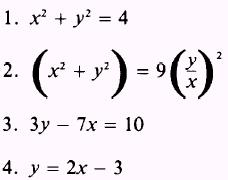Change the equations in the following problems to equations having Cartesian coordinates.ANSWERS: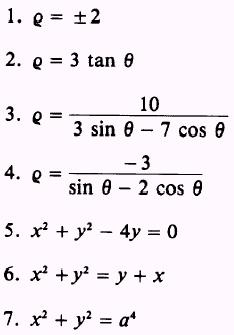SUMMARY The following are the major topics covered in this chapter: 1. Conic section: A conic section is the locus of all points in a plane whose distance from a fixed point is a constant ratio to its distance from a fixed line. The fixed point is the focus, and the fixed line is the directrix. The ratio referred to is called the eccentricity. 2. Eccentricity: If 0 < e < l, then the curve is an ellipse. If e > 1, then the curve is a hyperbola. If e = 1, then the curve is a parabola. If e = 0, then the curve is a circle. 3. Locus of an equation: The locus of an equation is a curve containing those points, and only those points, whose coordinates satisfy the equation. 4. Circle: A circle is the locus of all points, in a plane that is always a fixed distance, called the radius, from a fixed point, called the center. Theorem: An equation of the second degree, in which the coefficients of the x2 and y2 terms are equal and the xy term does not exist represents a circle. 5. Standard equation of a circle:where (x,y) is a point on the circle, (h,k) is the center, and r is the radius of the circle. 6. General equation of a circle: x2+y2+ Bx+Cy+D=O where B, C, and D are constants. 7. Circle defined by three points: A circle may be defined by three noncollinear points; that is, by three points not lying on 2-54 a straight line. Only one circle is possible through any three noncollinear points. To find the equation of the circle determined by three points, substitute the x and y values of each of the given points into the general equation to form three equations with B, C, and D as the unknowns. These equations are then solved simultaneously to find the values of B, C, and D in the equation that satisfies the three given conditions. 8. Parabola: A parabola is the locus of all points in a plane equidistant from a fixed point, called the focus, and a fixed line, called the directrix. The point which lies halfway between the focus and the directrix is called the vertex. The focal chord is equal to 4a, where a is the distance from the vertex to the focus. A parabola with its vertex at the origin and opening to the right has its focus at (a,0) and its directrix at x = -a; its corresponding equation is y2 = 4ax. A parabola with its vertex at the origin and opening to the left has its focus at ( - a,0) and its directrix at x = a; its corresponding equation is y2 = - 4ax. A parabola with its vertex at the origin and opening upward has its focus at (0,a) and its directrix at y = -a; its corresponding equation is x2 = 4ay. A parabola with its vertex at the origin and opening downward has its focus at (0, - a) and its directrix at y = a; its corresponding equation is x2 = - 4ay. 9. Standard equations for parabolas: 1. (y - k) 2 = 4a(x - h) (parabola opening to the right) 2. (y - k)2 = -4a(x - h) (parabola opening to the left) 3. (x - h)2 = 4a(y - k) (parabola opening upward) 4. (x - h)2 = - 4a(y - k) (parabola opening downward) where (h,k) is the coordinate of the vertex and a is the distance from the vertex to the focus. 10. Ellipse: An ellipse is the locus of all points, in a plane the sum of whose distances from two fixed points (the foci) is a constant equal to 2a. The ellipse is symmetrical with respect to the X and Y axes, so an ellipse with its center at the origin and its major axis along the X axis has foci at (� ae,0) or (� c,0), vertices at (� a,0), and directrices at x = �a/e; its corresponding equation is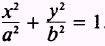. An ellipse with its center at the origin and its major axis along the Y axis has foci at (0, � ae) or (0, � c), vertices at (0, � a), and directrices at y = � a/e; its corresponding equation is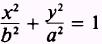. The distance from the center through the focus to the curve is always designated by a and is called the semimajor axis. The perpendicular distance from the midpoint of the major axis to the curve is called the semiminor axis and is always signified by b. The distance from the center of the ellipse to the focus is called c. The eccentricity is designated by e, which is equal to c/a. The following relationships are true for a, b, and c in an ellipse: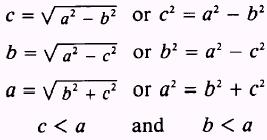Theorem: A"I'n equation of the second degree, in which the xy term does not exist and the coeficients of x2 and y2 are different but have the same sign, represents an ellipse with axes parallel to the coordinate axes. 11. Standard equations for ellipses:where (h,k) is the center of the ellipse, a is the length of the semimajor axis, and b is the length of the semiminor axis. 12. General equation of an ellipse: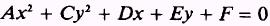where the capital letters refer to independent constants and A and C have the same sign. 13. Hyperbola: A hyperbola is the locus of all points in a plane such that the difference of their distances from two fixed points is constant. The fixed points are the foci and the constant difference is 2a. The transverse axis is of length 2a and is the distance between the intersections (vertices) of the hyperbola with its focal axis. The conjugate axis is of length 2b and is perpendicular to the transverse axis. The focal chord of a hyperbola is equal to. A hyperbola with its center at the origin and transverse axis along the X axis has foci at (� ae,0) or (� c,0), vertices at ( � a,0), directrices at x = � a/e, and asymptotes at y = � (b/a)x; its corresponding equation is. A hyperbola with its center at the origin and transverse axis along the Y axis has foci at (0, � ae) or (0, � c), vertices at (0, � a), directrices at y = � ale, and asymptotes at x = �(b/a)y; its corresponding equation is. The following relationships are true for a, b, and c in a hyperbola: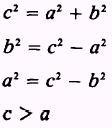14. Standard equations for hyperbolas: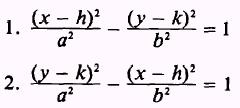where (h,k) is the center of the hyperbola, a is half the length of the transverse axis, and b is half the length of the conjugate axis. 15. General equation of a hyperbola: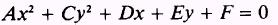where the capital letters refer to independent constants and A and C have different signs. 16. Polar coordinates: The position of any point, P, is fully determined if we know the directed distance, called the radius vector, and the angle that the radius vector makes with the reference line, called the polar angle. The radius vector is denoted by Q, while 0 denotes the polar angle. The origin is also called the pole. The polar angle is positive when measured counterclockwise and negative when measured clockwise. The radius vector is positive only when it is laid off on the terminal side of the angle. When the radius vector is laid off on the terminal side of the ray produced beyond the pole (the given angle plus 180% a negative value is assigned the radius vector. 17. Transformation from Cartesian to polar coordinates:18. Transformation from polar to Cartesian coordinates: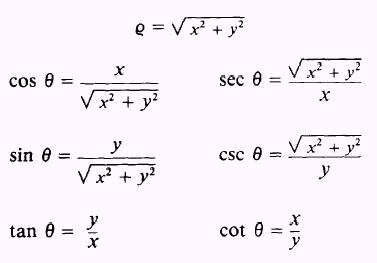Integrated Publishing, Inc. - A (SDVOSB) Service Disabled Veteran Owned Small Business